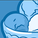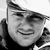Announcements
Product Release Webinar: Qlik Insider airing December 6! REGISTER TODAY!
cancel
Showing results for
Did you mean:Contributor III

## Qlikview Sum to Higher Level Dimension

Hello,

Please see the attached screenshot providing details of the below question.

I currently have a table at a lower dimension of States representing tickets and weeks in two different columns. For each state (row), I multiply the #of tickets by the # of weeks to get a total. I then have a sum of at the top.

My question is, how can I get the same totals at a higher level dimension (Country)?

Tickets =  Sum(Tickets)

WKS = Sum(WKS)

Tickets x WKS = Sum(Tickets) x Sum(WKS)

Since in the second table I am removing the dimension of State, all that is left is one row with one summed value of tickets, and one summed value of WKS. Multiplying those two values however, produces a sum that is larger than expected output.

Any help is greatly appreciated.

Regards

Labels (4)

• ### SUM IFS

3 RepliesMVP
It sounds to me like you are looking for an average Sum(Tickets) x Sum(WKS) per some kind of dimension combination. E.g. Colour and week. To accomplish that you will need to use the aggr functionality.

AVG(AGGR(Sum(Tickets) x Sum(WKS), Color, WKS))MVP

I would also recommend you to look at the alternative KPI tickets per week.

Sum(Tickets) / Sum(WKS)

This expression will scale well even when changing to higher level dimensions.Contributor III
Author

Hi Vegar,

Thank you for your help, again!

While your suggestions did not directly provide the solution to my problem, they better helped me understand what I am trying to do, as well as the exact problem I am running into. That being the case, I opened a separate question on my problem here: https://community.qlik.com/t5/New-to-QlikView/Taking-the-Sum-of-an-already-summed-expression/m-p/156....

Thank you againCommunity Browser